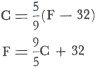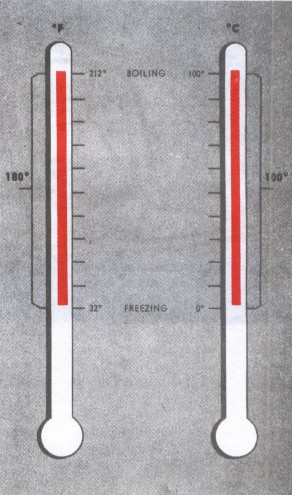# Aviation Weather

full text of the classic FAA guide

## TEMPERATURE SCALES

Two commonly used temperature scales are Celsius (Centigrade) and Fahrenheit. The Celsius scale is used exclusively for upper air temperatures and is rapidly becoming the world standard for surface temperatures also.

Traditionally, two common temperature references are the melting point of pure ice and the boiling point of pure water at sea level. The melting point of ice is 0° C or 32° F; the boiling point of water is 100° C or 212° F. Thus, the difference between melting and boiling is 100 degrees Celsius or 180 degrees Fahrenheit; the ratio between degrees Celsius and Fahrenheit is 100/180 or 5/9. Since 0° F is 32 Fahrenheit degrees colder than 0° C, you must apply this difference when comparing temperatures on the two scales. You can convert from one scale to the other using one of the following formulae:where C is degrees Celsius and F is degrees Fahrenheit. Figure 3 compares the two scales. Many flight computers provide for direct conversion of temperature from one scale to the other. Section 16, AVIATION WEATHER SERVICES has a graph for temperature conversion.

Temperature we measure with a thermometer. But what makes a thermometer work? Simply the addition or removal of heat. Heat and temperature are not the same; how are they related?FIGURE 3. The two temperature scales in common use are the Fahrenheit and the Celsius. 9 degrees on the Fahrenheit scale equal 5 degrees on the Celsius.

A PDF version of this book is available here. You may be able to buy a printed copy of the book from amazon.com.